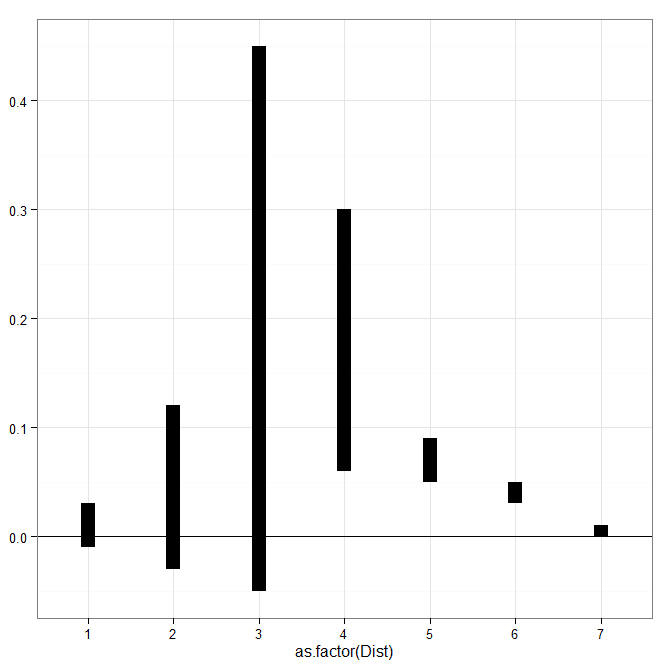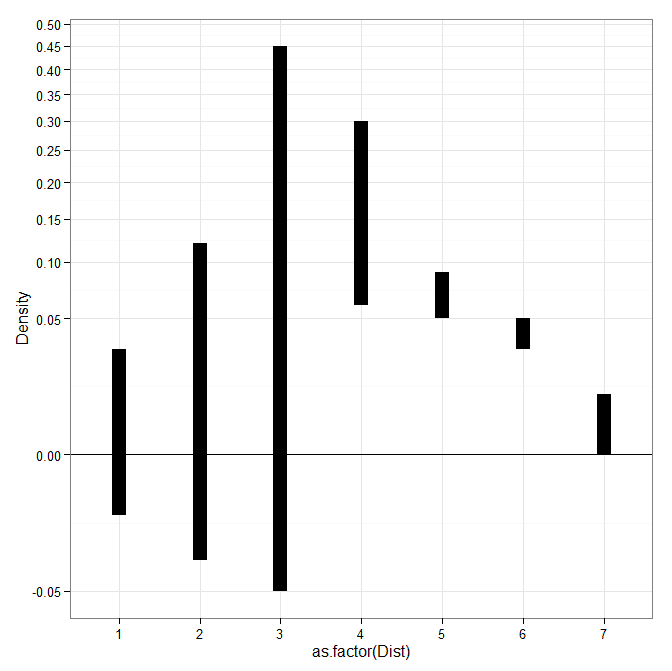# Custom square root scale (with negative values) in ggplot2 (R)

My prior rootogram post Jon Peck made the astute comment that rootograms typically are plotted on a square root scale. (Which should of been obvious to me given the name!) The reason for a square root scale for rootograms is visualization purposes, the square root scale gives more weight to values nearby 0 and shrinks values farther away from 0.

SPSS can not have negative values on a square root scale, but you can make a custom scale using ggplot2 and the scales package in R for this purpose. Here I just mainly replicated this short post by Paul Hiemstra.

So in R, first we load the `scales` and the `ggplot2` package, and then create our custom scale function. Obviously the square root of a negative value is not defined for real numbers, so what we do is make a custom square root function. The function simply takes the square root of the absolute value, and then multiplies by the sign of the original value. This function I name `S_sqrt` (for signed square root). We also make its inverse function, which is named `IS_sqrt`. Finally I make a third function, `S_sqrt_trans`, which is the one used by the scales package.

``````library(scales)
library(ggplot2)

S_sqrt <- function(x){sign(x)*sqrt(abs(x))}
IS_sqrt <- function(x){x^2*sign(x)}
S_sqrt_trans <- function() trans_new("S_sqrt",S_sqrt,IS_sqrt)``````

Here is a quick example data set in R to work with.

``````#rootogram example, see http://stats.stackexchange.com/q/140473/1036
MyText <- textConnection("
Dist Val1 Val2
1 0.03 0.04
2 0.12 0.15
3 0.45 0.50
4 0.30 0.24
5 0.09 0.04
6 0.05 0.02
7 0.01 0.01
")
MyData\$Hang <- MyData\$Val1 - MyData\$Val2``````

And now we can make our plots in `ggplot2`. First the linear scale, and second update our plot to the custom square root scale.

``````p <- ggplot(data=MyData, aes(x = as.factor(Dist), ymin=Hang, ymax=Val1)) +
geom_hline(aes(yintercept=0)) + geom_linerange(size=5) + theme_bw()
p

p2 <- p + scale_y_continuous(trans="S_sqrt",breaks=seq(-0.1,0.5,0.05), name="Density")
p2``````### 1 Comment

1.